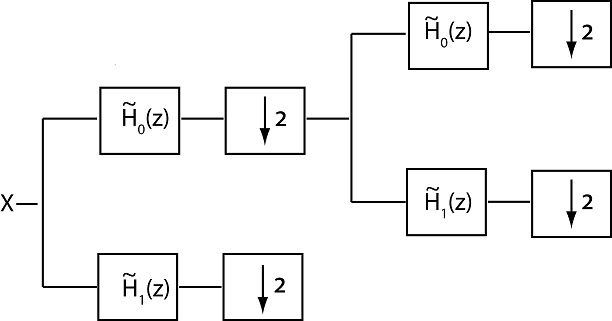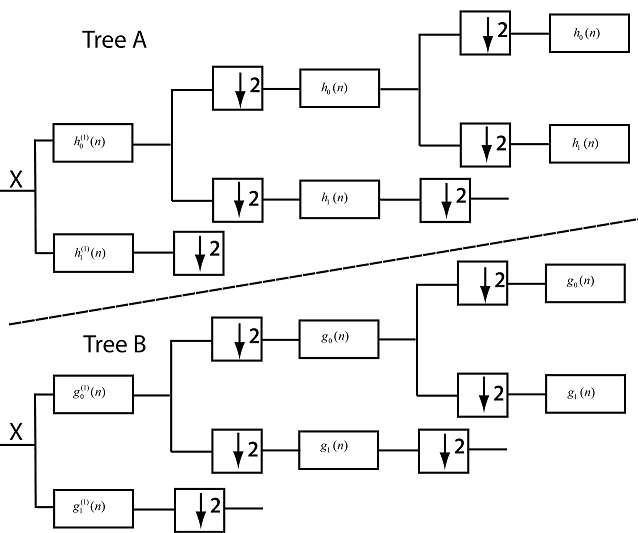## Critically Sampled and Oversampled Wavelet Filter Banks

Wavelet filter banks are special cases of multirate filter banks called tree-structured filter banks. In a filter bank, two or more filters are applied to an input signal and the filter outputs are typically downsampled. The following figure illustrates two stages, or levels, of a critically sampled two-channel tree-structured analysis filter bank. The filters are depicted in the z domain.The filter system functions, ${\stackrel{˜}{H}}_{0}\left(z\right)$ and ${\stackrel{˜}{H}}_{1}\left(z\right)$, are typically designed to approximately partition the input signal, X, into disjoint subbands. In wavelet tree-structured filter banks, the filter ${\stackrel{˜}{H}}_{0}\left(z\right)$ is a lowpass, or scaling, filter, with a non-zero frequency response on the interval [-π/2, π/2] radians/sample or [-1/4, 1/4] cycles/sample. The filter ${\stackrel{˜}{H}}_{1}\left(z\right)$ is a highpass, or wavelet, filter, with a non-zero frequency response on the interval [-π, -π/2] ∪ [π/2, π] radians/sample or [-1/2, -1/4] ∪ [1/4, 1/2] cycles/sample. The filter bank iterates on the output of the lowpass analysis filter to obtain successive levels resulting into an approximate octave-band filtering of the input. The two analysis filters are not ideal, which results in aliasing that must be canceled by appropriately designed synthesis filters for perfect reconstruction. For an orthogonal filter bank, the union of the scaling filter and its even shifts and the wavelet filter and its even shifts forms an orthonormal basis for the space of square-summable sequences, ${\ell }^{2}\left(ℤ\right)$. The synthesis filters are the time-reverse and conjugates of the analysis filters. For biorthogonal filter banks, the synthesis filters and their even shifts form the reciprocal, or dual, basis to the analysis filters. With two analysis filters, downsampling the output of each analysis filter by two at each stage ensures that the total number of output samples equals the number of input samples. The case where the number of analysis filters is equal to the downsampling factor is referred to as critical sampling. An analysis filter bank where the number of channels is greater than the downsampling factor is an oversampled filter bank.

### Double-Density Wavelet Transform

The following figure illustrates two levels of an oversampled analysis filter bank with three channels and a downsampling factor of two. The filters are depicted in the z domain.Assume the filter ${\stackrel{˜}{H}}_{0}\left(z\right)$, is a lowpass half-band filter and the filters ${\stackrel{˜}{H}}_{1}\left(z\right)$ and ${\stackrel{˜}{H}}_{2}\left(z\right)$ are highpass half-band filters.

Assume the three filters together with the corresponding synthesis filters form a perfect reconstruction filter bank. If additionally, ${\stackrel{˜}{H}}_{1}\left(z\right)$ and ${\stackrel{˜}{H}}_{2}\left(z\right)$ generate wavelets that satisfy the following relation

${\psi }_{1}\left(t\right)={\psi }_{2}\left(t-1/2\right),$

the filter bank implements the double-density wavelet transform. The preceding condition guarantees that the integer translates of one wavelet fall halfway between the integer translates of the second wavelet. In frame-theoretic terms, the double-density wavelet transform implements a tight frame expansion.

The following code illustrates the two wavelets used in the double-density wavelet transform.

x = zeros(256,1); df = dtfilters('filters1'); wt1 = dddtree('ddt',x,5,df,df); wt2 = dddtree('ddt',x,5,df,df); wt1.cfs{5}(5,1,1) = 1; wt2.cfs{5}(5,1,2) = 1; wav1 = idddtree(wt1); wav2 = idddtree(wt2); plot(wav1); hold on; plot(wav2,'r'); axis tight; legend('\psi_1(t)','\psi_2(t)')

You cannot choose the two wavelet filters arbitrarily to implement the double-density wavelet transform. The three analysis and synthesis filters must satisfy the perfect reconstruction (PR) conditions. For three real-valued filters, the PR conditions are

$\begin{array}{l}{H}_{0}\left(z\right){H}_{0}\left(1/z\right)+{H}_{1}\left(z\right){H}_{1}\left(1/z\right)+{H}_{2}\left(z\right){H}_{2}\left(1/z\right)=2\\ {H}_{0}\left(z\right){H}_{0}\left(-1/z\right)+{H}_{1}\left(z\right){H}_{1}\left(-1/z\right)+{H}_{2}\left(z\right){H}_{2}\left(-1/z\right)=0\end{array}$

You can obtain wavelet analysis and synthesis frames for the double-density wavelet transform with 6 and 12 taps using dtfilters.

[df1,sf1] = dtfilters('filters1'); [df2,sf2] = dtfilters('filters2');

df1 and df2 are three-column matrices containing the analysis filters. The first column contains the scaling filter and columns two and three contain the wavelet filters. The corresponding synthesis filters are in sf1 and sf2.

See  and  for details on how to generate wavelet frames for the double-density wavelet transform.

The main advantages of the double-density wavelet transform over the critically sampled discrete wavelet transform are

• Reduced shift sensitivity

• Reduced rectangular artifacts in the 2-D transform

• Smoother wavelets for a given number of vanishing moments

• Increased computational costs

• Non-orthogonal transform

Additionally, while exhibiting less shift sensitivity than the critically sampled DWT, the double-density DWT is not shift-invariant like the complex dual-tree wavelet transform. The double-density wavelet transform also lacks the directional selectivity of the oriented dual-tree wavelet transforms.

### Dual-Tree Complex Wavelet Transform

The critically sampled discrete wavelet transform (DWT) suffers from a lack of shift invariance in 1-D and directional sensitivity in N-D. You can mitigate these shortcomings by using approximately analytic wavelets. An analytic wavelet is defined as

${\psi }_{c}\left(t\right)={\psi }_{r}\left(t\right)+j{\psi }_{i}\left(t\right)$

where j denotes the unit imaginary. The imaginary part of the wavelet, ψi(t), is the Hilbert transform of the real part, ψr(t). In the frequency domain, the analytic wavelet has support on only one half of the frequency axis. This means that the analytic wavelet ψc(t) has only one half the bandwidth of the real-valued wavelet ψr(t).

It is not possible to obtain exactly analytic wavelets generated by FIR filters. The Fourier transforms of compactly supported wavelets cannot vanish on any set of nonzero measure. This means that the Fourier transform cannot be zero on the negative frequency axis. Additionally, the efficient two-channel filter bank implementation of the DWT derives from the following perfect reconstruction condition for the scaling filter, ${H}_{0}\left({e}^{j\omega }\right)$, of a multiresolution analysis (MRA)

$|{H}_{0}\left({e}^{j\omega }\right){|}^{2}+|{H}_{0}\left({e}^{j\left(\omega +\pi \right)}\right){|}^{2}=2.$

If the wavelet associated with an MRA is analytic, the scaling function is also analytic. This implies that

${H}_{0}\left({e}^{j\omega }\right)=0\text{ }-\pi \le \omega <0,$

from which it follows that $|{H}_{0}\left({e}^{j\omega }\right){|}^{2}=2\text{ }0\le \omega \le \pi .$ The result is that the scaling filter is allpass.

The preceding results demonstrate that you cannot find a compactly support wavelet determined by FIR filters that is exactly analytic. However, you can obtain wavelets that are approximately analytic by combining two tree-structured filter banks as long as the filters in the dual-tree transform are carefully constructed to satisfy certain conditions ,.

The dual-tree complex wavelet transform is implemented with two separate two-channel FIR filter banks. The output of one filter bank is considered to be the real part, while the output of the other filter bank is the imaginary part. Because the dual-tree complex wavelet transform uses two critically sampled filter banks, the redundancy is 2d for a d-dimensional signal (image). There are a few critical considerations in implementing the dual-tree complex wavelet transform. For convenience, refer to the two trees as: Tree A and Tree B.

• The analysis filters in the first stage of each filter bank must differ from the filters used at subsequent stages in both trees. It is not important which scaling and wavelet filters you use in the two trees for stage 1. You can use the same first stage scaling and wavelet filters in both trees.

• The scaling filter in Tree B for stages ≥ 2 must approximate a 1/2 sample delay of the scaling filter in Tree A. The one-half sample delay condition is a necessary and sufficient condition for the corresponding Tree B wavelet to be the Hilbert transform of the Tree A wavelet..

The following figure illustrates three stages of the analysis filter bank for the 1-D dual-tree complex wavelet transform. The FIR scaling filters for the two trees are denoted by $\left\{{h}_{0}\left(n\right),\text{ }\text{\hspace{0.17em}}{g}_{0}\left(n\right)\right\}$. The FIR wavelet filters for the two trees are denoted by $\left\{{h}_{1}\left(n\right),{g}_{1}\left(n\right)\right\}$. The two scaling filters are designed to approximately satisfy the half-sample delay condition

${g}_{0}\left(n\right)={h}_{0}\left(n-1/2\right)$

The superscript (1) denotes that the first-stage filters must differ from the filters used in subsequent stages. You can use any valid scaling-wavelet filter pair for the first stage. The filters $\left\{{h}_{0}\left(n\right),\text{ }\text{\hspace{0.17em}}{g}_{0}\left(n\right)\right\}$ cannot be arbitrary scaling filters and provide the benefits of using approximately analytic wavelets.#### 2-D Dual-Tree Wavelet Transforms

The dual-tree wavelet transform with approximately analytic wavelets offers substantial advantages over the separable 2-D DWT for image processing. The traditional separable 2-D DWT suffers from checkerboard artifacts due to symmetric frequency support of real-valued (non-analytic) scaling functions and wavelets. Additionally, the critically sampled separable 2-D DWT lacks shift invariance just as the 1-D critically sampled DWT does. The Wavelet Toolbox™ software supports two variants of the dual-tree 2-D wavelet transform, the real oriented dual-tree wavelet transform and the oriented 2-D dual-tree complex wavelet transform. Both are described in detail in .

The real oriented dual-tree transform consists of two separable (row and column filtering) wavelet filter banks operating in parallel. The complex oriented 2-D wavelet transform requires four separable wavelet filter banks and is therefore not technically a dual-tree transform. However, it is referred to as a dual-tree transform because it is the natural extension of the 1-D complex dual-tree transform. To implement the real oriented dual-tree wavelet transform, use the 'realdt' option in dddtree2. To implement the oriented complex dual-tree transform, use the 'cplxdt' option.

Both the real oriented and oriented complex dual-tree transforms are sensitive to directional features in an image. Only the oriented complex dual-tree transform is approximately shift invariant. Shift invariance is not a feature possessed by the real oriented dual-tree transform.

### Dual-Tree Double-Density Wavelet Transforms

The dual-tree double-density wavelet transform combines the properties of the double-density wavelet transform and the dual-tree wavelet transform .

In 1-D, the dual-tree double-density wavelet transform consists of two three-channel filter banks. The two wavelets in each tree satisfy the conditions described in Double-Density Wavelet Transform. Specifically, the integer translates of one wavelet fall halfway between the integer translates of the second wavelet. Additionally, the wavelets in Tree B are the approximate Hilbert transform of the wavelets in Tree A. To implement the dual-tree double-density wavelet transform for 1-D signals, use the 'cplxdddt' option in dddtree. Similar to the dual-tree wavelet transform, the dual-tree double-density wavelet transform provides both real oriented and complex oriented wavelet transforms in 2-D. To obtain the real oriented dual-tree double-density wavelet transform, use the 'realdddt' option in dddtree2. To obtain the complex oriented dual-tree double-density wavelet transform, use the 'cplxdddt' option.

 Kingsbury, N.G. “Complex Wavelets for Shift Invariant Analysis and Filtering of Signals”. Journal of Applied and Computational Harmonic Analysis. Vol 10, Number 3, May 2001, pp. 234-253.

 Selesnick, I. “The Double-Density Dual-Tree Wavelet Transform”. IEEE® Transactions on Signal Processing. Vol. 52, Number 5, May 2004, pp. 1304–1314.

 Selesnick, I. “The Design of Approximate Hilbert Transform Pairs of Wavelet Bases.” IEEE Transactions on Signal Processing, Vol. 50, Number 5, pp. 1144–1152.

 Selesnick, I. “The Double Density DWT” Wavelets in Signal and Image Analysis: From Theory to Practice (A.A Petrosian, F.G. Meyer, eds.). Norwell, MA: Kluwer Academic Publishers:, 2001.

 Abdelnour, F. “Symmetric Wavelets Dyadic Siblings and Dual Frames” Signal Processing, Vol. 92, Number 5, 2012, pp. 1216–1225.

 Selesnick, I,. R.G Baraniuk, and N.G. Kingsbury. “The Dual-Tree Complex Wavelet Transform.” IEEE Signal Processing Magazine. Vol. 22, Number 6, November, 2005, pp. 123–151.

 Vetterli, M. “Wavelets, Approximation, and Compression”. IEEE Signal Processing Magazine, Vol. 18, Number 5, September, 2001, pp. 59–73.